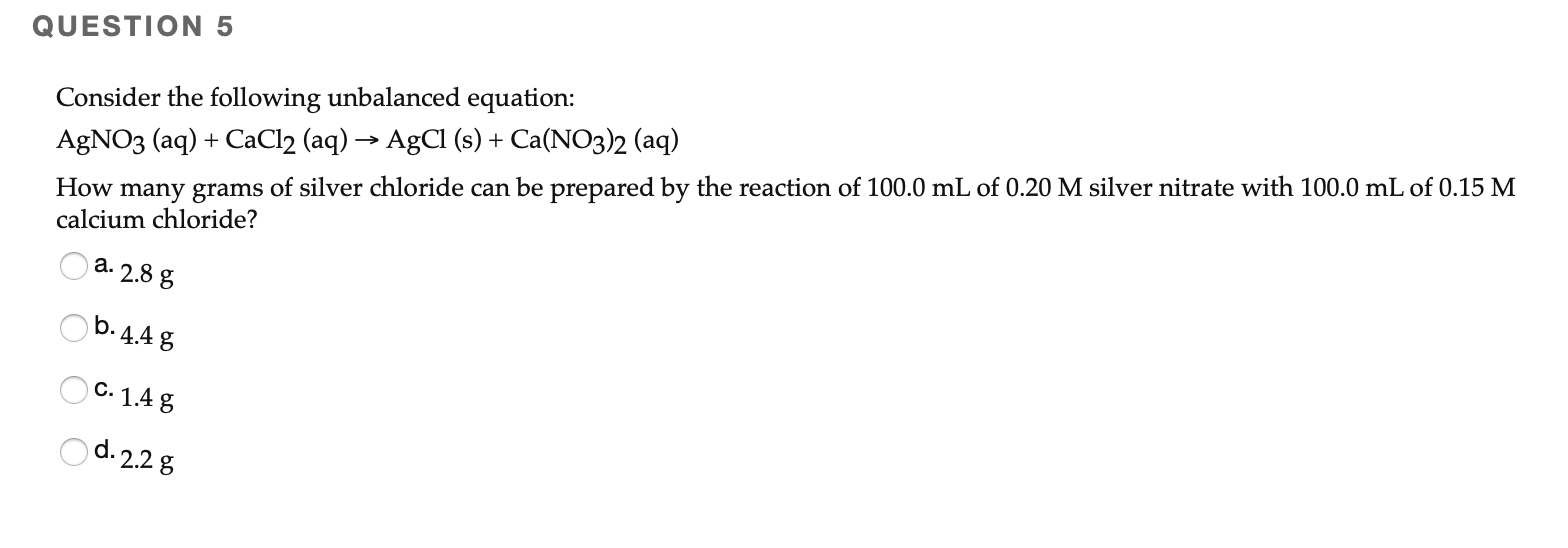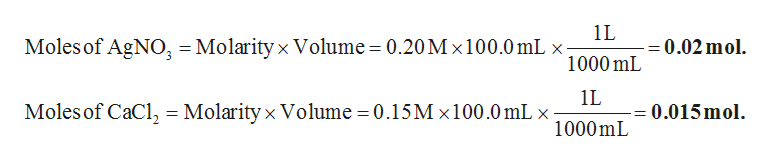# QUESTION 5Consider the following unbalanced equation:How many grams of silver chloride can be prepared by the reaction of 100.0 mL of 0.20 M silver nitrate with 100.0 mL of 0.15 Mcalcium chloride?AgNO3 (aq) + CaCl2 (aq) → AgCl (s) + Ca(NO3)2 (aq)a. 2.8 gOb. 4.4 gO C. 1.4 gO d. 2.2 g

Question
5 views

problem in photo attachedhelp_outlineImage TranscriptioncloseQUESTION 5 Consider the following unbalanced equation: How many grams of silver chloride can be prepared by the reaction of 100.0 mL of 0.20 M silver nitrate with 100.0 mL of 0.15 M calcium chloride? AgNO3 (aq) + CaCl2 (aq) → AgCl (s) + Ca(NO3)2 (aq) a. 2.8 g Ob. 4.4 g O C. 1.4 g O d. 2.2 g fullscreen
check_circle

Step 1

The balanced chemical equation is given below.

Step 2

The actual moles of silver nitrate and calcium chloride can be calculated as given below.help_outlineImage Transcriptionclose1L Moles of AgNO, = Molarity x Volume = 0.20M x100.0 mL x = 0.02 mol. 1000 mL IL Moles of CaCl, = Molarity x Volume = 0.15M x100.0 mL x 0.015 mol. %3D 1000 mL fullscreen
Step 3

The molar ratio of AgCl and silver nitrate is 1:1. Therefore, the number of moles of AgCl produced from 0.02 moles of silver nitrate is 0.02 mol.

The molar ratio of AgCl and calcium chloride is 2:1. Therefor...

### Want to see the full answer?

See Solution

#### Want to see this answer and more?

Solutions are written by subject experts who are available 24/7. Questions are typically answered within 1 hour.*

See Solution
*Response times may vary by subject and question.
Tagged in

### Physical Chemistry